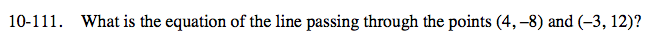### Home > CCA > Chapter 10 > Lesson 10.3.1 > Problem10-111

10-111.

What is the equation of the line passing through the points (4, –8) and (–3, 12)? Homework Help ✎Use the two points to find the slope of the line. Then use y = mx + b and one of the points to find b.

$y=-\frac{20}{7} x+\frac{24}{7}$